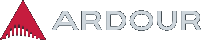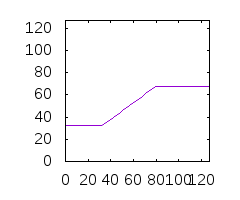# Midi velocity curve correction plugin

Hi
Can you guys recommend a plugin (linux native preferably) for midi velocity curve correction?
I’m only aware of Robin’s “Midi Velocity Adjust” but as far as I understand it only lets you adjust Min and Max velocity values - nothing in between.

Ok, so maybe it could be done via lua script? Something like:
if incoming midi velocity is in the range of 60-80 then convert it to 70
if incoming midi velocity is in the range of 80-100 then convert it to 90
etc
etc
Could it be easily done? If so I would be really grateful for any hints how to write it.

The “Midi Velocity Adjust” does linear interpolation of the values in the target-range for example to 32…68 (no offset) the mapping would be as depicted below (x-axis is input velocity, y-axis output):It does however not offer control over the the curve and certainly not a piecewise function, but yes, you can do that with a Lua script: save the following to your config GNU/Linux: `~/.config/ardour5/scripts/mscale.lua` and edit the function `map_velocity` as needed.

``````ardour {
["type"]    = "dsp",
name        = "MIDI Velcocity Hack",
category    = "Example", -- "Utility"
author      = "Ardour Lua Task Force",
description = [[An Example Midi Filter for prototyping.]]
}

function dsp_ioconfig ()
return { { midi_in = 1, midi_out = 1, audio_in = 0, audio_out = 0}, }
end

function dsp_run (_, _, n_samples)
local cnt = 1;
function tx_midi (time, data)
midiout[cnt] = {}
midiout[cnt]["time"] = time;
midiout[cnt]["data"] = data;
cnt = cnt + 1;
end

function map_velocity (v)
if v < 50 then
return 1 -- min velocity for a note-on event is 1
elseif v < 70 then -- 50 .. 69
return 50
elseif v < 90 then -- 70 ..89
return 80
else               -- 90 .. 127
return 90 + math.floor (25 * ((v - 90) / 37)) -- maps linearly to 90..115
end
end

-- for each incoming midi event
for _,b in pairs (midiin) do
local t = b["time"] -- t = [ 1 .. n_samples ]
local d = b["data"] -- get midi-event bytes
local event_type
if #d == 0 then event_type = -1 else event_type = d >> 4 end
if (#d == 3 and event_type == 9) then -- note on
d = map_velocity (d)
tx_midi (t, d)
else -- pass thru all other events unmodified
tx_midi (t, d)
end
end
end
``````

It is based on two existing script that may also provide further insights

Yes! Ardour Lua Task Force to the rescue againThank you so much Robin!

For a simple “rounding”, the MIDI Transform tool can be enough, with something like:

``````Set [Velocity] to [this note's] [velocity]
/ [exactly] 20
* [exactly] 20
+ [exactly] 10
``````

This will map [0 - 20[ to 10, [20 - 40[ to 30 etc…

1 Like

PS. I do recall seeing a plugin that allows to draw curves, exponential mappings and such, similar to pianoteq’s built-in, but I was not able to find it anymore. In case someone happens stumbles upon this, I’d be interested as well.

Nice trick, I didn’t know that one. Thank you!

Maybe at one point I’ll be tricked into writing a “gamma” lua dsp plugin with an inline UI…

I’ve made a quick hack base on @x42 's example code and implemented midi velocity curve correction, briefly tested, seems worked.

Can you please give some hints about how to make the velocity curve configurable?

Note that I’m not a pro coder, and know little about Ardour. Just started using Ardour a week ago.

``````ardour {
["type"]    = "dsp",
name        = "MIDI Velcocity Curve",
category    = "Utility",
author      = "Ardour Lua Task Force",
description = [[Midi Filter for Velocity Curve.]]
}

function dsp_ioconfig ()
return { { midi_in = 1, midi_out = 1, audio_in = 0, audio_out = 0}, }
end

function dsp_run (_, _, n_samples)
local cnt = 1;
function tx_midi (time, data)
midiout[cnt] = {}
midiout[cnt]["time"] = time;
midiout[cnt]["data"] = data;
cnt = cnt + 1;
end

local mapx = {10, 95, 105, 116, 127}
local mapy = {0, 105, 116, 123, 127}
function map_velocity (v)
local i = v // (127 // (#mapx - 1)) + 1
while true do
if i <= 0 then
return mapy
end
if v >= mapx[i] then
if i >= #mapx then
return mapy[i]
end
if v < mapx[i+1] then
return mapy[i] + math.floor((v - mapx[i]) * (mapy[i+1] - mapy[i]) / (mapx[i+1] - mapx[i]))
else
i = i + 1
end
else
i = i - 1
end
end
end

-- for each incoming midi event
for _,b in pairs (midiin) do
local t = b["time"] -- t = [ 1 .. n_samples ]
local d = b["data"] -- get midi-event bytes
local event_type
if #d == 0 then event_type = -1 else event_type = d >> 4 end
if (#d == 3 and event_type == 9) then -- note on
d = map_velocity (d)
tx_midi (t, d)
else -- pass thru all other events unmodified
tx_midi (t, d)
end
end
end

``````

Hi guys !

I’m such a big fan of Ardour !
Had the same need and fell into this nice post !
I got inspired and improved the code above to implement linear, exponential and logarithmic curves with customized slope. I know it could be improved but I thought it would be nice to share it to the community !

EDIT : I improved the plugin by adding a note filter so that the curves would only apply to specific notes. And I finally created a Github repository for this script. See here : https://github.com/remyzerems/MIDI-Velocity-Curve

``````ardour {
["type"]    = "dsp",
name        = "MIDI Velocity Curve",
category    = "Utility",
author      = "Ardour Lua Task Force",
description = [[Midi Filter for Velocity Curve.]]
}

function dsp_ioconfig ()
return { { midi_in = 1, midi_out = 1, audio_in = 0, audio_out = 0}, }
end

function dsp_params ()
return
{
{ ["type"] = "input", name = "Curve", min = 0, max = 2, default = 0, enum = true, scalepoints =
{
["Linear"]    = 0,
["Logarithmic"]  = 1,
["Exponential"] = 2,
}
},
{ ["type"] = "input", name = "Slope", min = 0.001, max = 10,    default = 1,    unit="" }
}
end

function dsp_run (_, _, n_samples)
local cnt = 1;
function tx_midi (time, data)
midiout[cnt] = {}
midiout[cnt]["time"] = time;
midiout[cnt]["data"] = data;
cnt = cnt + 1;
end

function check_limits(v)
if v < 1 then
v = 1
elseif v > 127 then
v = 127
end
return math.floor (v)
end

function linear_curve(v, slope)
return check_limits ( slope*v )
end

function log_curve (v, slope)
return check_limits ( 127*math.log(slope*v)/math.log(slope*127) )
end

function exp_curve (v, slope)
return check_limits ( 127*(math.exp(slope*v/127)-1)/(math.exp(slope)-1) )
end

function map_velocity (v)
local ctrl = CtrlPorts:array ()
local slope = ctrl
if ctrl == 1 then
return log_curve (v, slope)
elseif ctrl == 2 then
return exp_curve (v, slope)
else
return linear_curve (v, slope)
end
end

-- for each incoming midi event
for _,b in pairs (midiin) do
local t = b["time"] -- t = [ 1 .. n_samples ]
local d = b["data"] -- get midi-event bytes
local event_type
if #d == 0 then event_type = -1 else event_type = d >> 4 end
if (#d == 3 and event_type == 9) then -- note on
d = map_velocity (d)
tx_midi (t, d)
else -- pass thru all other events unmodified
tx_midi (t, d)
end
end
end``````
2 Likes

@x42 Is the InserPizHere MidiCurve for velocity or any CC (he has MidiPitchBendCurve too), many of their plugins are ported to Linux (the no-GUI plugins) but Juce-gui plugins don’t.

The code is on google yet: